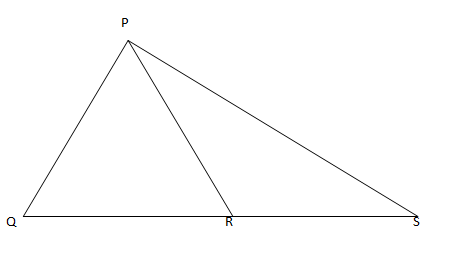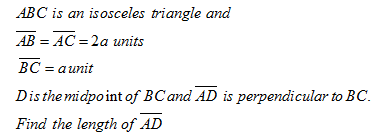• This is an assessment test.
• These tests focus on geometry and mensuration and are meant to indicate your preparation level for the subject.
• Kindly take the tests in this series with a pre-defined schedule.

## Geometry and Mensuration: Test 20

Congratulations - you have completed Geometry and Mensuration: Test 20. You scored %%SCORE%% out of %%TOTAL%%. You correct answer percentage: %%PERCENTAGE%% . Your performance has been rated as %%RATING%%
 Question 1
The side QR of an equilateral triangle PQR is produced to the point S in such a way that QR= RS and P is joined to S. Then the measure of ∠PSR is
 A 30o B 15o C 60o D 45o
Question 1 Explanation:Here ∠PRQ = 600 so ∠PRS = 1200. Now QR = PR = RS.
Hence ∠PSR = ∠RPS
Now ∠PRS + ∠PSR + ∠RPS = 1800
=>2 ∠PSR = 1800 - 1200 = 600
=> ∠PSR = 300.
 Question 2
ABC is an isosceles triangle with AB= AC. A circle through B touching AC at the middle point intersects AB at P, Then AP: AB is:
 A 4: 1 B 2: 3 C 3: 5 D 1: 4
Question 2 Explanation:
$\displaystyle \begin{array}{l}\begin{array}{*{35}{l}} AM\text{ }is\text{ }the\text{ }tangent\text{ }to\text{ }the\text{ }circle\text{ }and \\ APB\text{ }is\text{ }a\text{ }secant\text{ }to\text{ }the\text{ }circle. \\ So~AM{}^\text{2}\text{ }=\text{ }AP\text{ }*\text{ }AB \\ \end{array}\\\begin{array}{*{35}{l}} But\text{ }M\text{ }is\text{ }the\text{ }mid-point\text{ }of\text{ }AC\text{ }and \\ AC\text{ }=\text{ }AB \\ Thus\text{ }AM\text{ }=\text{ }\left( 1/2 \right)AB \\ \end{array}\\AM{}^\text{2}\text{ }=\text{ }\frac{1}{4}AB{}^\text{2}\\\begin{array}{*{35}{l}} and\text{ }we\text{ }have\text{ }got\text{ }AM{}^\text{2}\text{ }=\text{ }AP\text{ }\times \text{ }AB \\ Hence,\text{ }AP:AB\text{ }=\text{ }1:4 \\ \end{array}\end{array}$
 Question 3A $\displaystyle \sqrt{15}\,\,a\,\,\,unit$ B $\displaystyle \frac{\sqrt{15}}{2}\,\,a\,\,unit$ C $\displaystyle \sqrt{17}\,\,a\,\,unit$ D $\displaystyle \frac{\sqrt{17}}{2}\,\,a\,\,unit$
Question 3 Explanation:$\begin{array}{l}\overline{AD}=\sqrt{{{(2a)}^{2}}-{{(\frac{a}{2})}^{2}}}\\=\frac{\sqrt{15}}{2}a\end{array}$
 Question 4
 A 30o B 60o C 45o D 65o
Question 4 Explanation:
$\begin{array}{l}\angle DCB={{60}^{o}}\\=>\angle DCE={{30}^{o}}\\Thus,\\\angle DEC=90-30={{60}^{o}}\end{array}$
 Question 5
The sum of interior angles of a regular polygon is 720o. The number of sides of the polygon is
 A 10 B 12 C 6 D 8
Question 5 Explanation:
$\displaystyle \begin{array}{l}If\text{ }the\text{ }number\text{ }of\text{ }sides\text{ }of\text{ }regular\text{ }polygon\text{ }be\text{ }n,\text{ }then\\\left( 2n-4 \right)\times {{90}^{o}}={{720}^{o}}\\\Rightarrow 2n-4=\frac{720}{90}=8\\\Rightarrow 2n-4=8\\\Rightarrow 2n=12\\\Rightarrow n=6\end{array}$
Once you are finished, click the button below. Any items you have not completed will be marked incorrect.
There are 5 questions to complete.
 ← List →